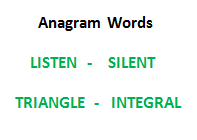# Python Program To Check Whether Two Strings Are Anagram Of Each Other

• Last Updated : 20 Dec, 2021

Write a function to check whether two given strings are anagram of each other or not. An anagram of a string is another string that contains the same characters, only the order of characters can be different. For example, “abcd” and “dabc” are an anagram of each other.## We strongly recommend that you click here and practice it, before moving on to the solution.

Method 1 (Use Sorting):

1. Sort both strings
2. Compare the sorted strings

Below is the implementation of the above idea:

## Python

 `# Python program to check whether two ``# strings are anagrams of each other`` ` `# Function to check whether two strings ``# are anagram of each other``def` `areAnagram(str1, str2):``    ``# Get lengths of both strings``    ``n1 ``=` `len``(str1)``    ``n2 ``=` `len``(str2)`` ` `    ``# If length of both strings is not ``    ``# same, then they cannot be anagram``    ``if` `n1 !``=` `n2:``        ``return` `0`` ` `    ``# Sort both strings``    ``str1 ``=` `sorted``(str1)``    ``str2 ``=` `sorted``(str2)`` ` `    ``# Compare sorted strings``    ``for` `i ``in` `range``(``0``, n1):``        ``if` `str1[i] !``=` `str2[i]:``            ``return` `0`` ` `    ``return` `1`` ` `# Driver code``str1 ``=` `"test"``str2 ``=` `"ttew"`` ` `# Function Call``if` `areAnagram(str1, str2):``    ``print``(``    ``"The two strings are anagram of each other"``)``else``:``    ``print``(``    ``"The two strings are not anagram of each other"``)``# This code is contributed by Bhavya Jain`

Output:

`The two strings are not anagram of each other`

Time Complexity: O(nLogn)

Method 2 (Count characters):
This method assumes that the set of possible characters in both strings is small. In the following implementation, it is assumed that the characters are stored using 8 bit and there can be 256 possible characters.

1. Create count arrays of size 256 for both strings. Initialize all values in count arrays as 0.
2. Iterate through every character of both strings and increment the count of character in the corresponding count arrays.
3. Compare count arrays. If both count arrays are same, then return true.

Below is the implementation of the above idea:

## Python

 `# Python program to check if two strings ``# are anagrams of each other``NO_OF_CHARS ``=` `256`` ` `# Function to check whether two strings ``# are anagram of each other``def` `areAnagram(str1, str2):`` ` `    ``# Create two count arrays and initialize ``    ``# all values as 0``    ``count1 ``=` `[``0``] ``*` `NO_OF_CHARS``    ``count2 ``=` `[``0``] ``*` `NO_OF_CHARS`` ` `    ``# For each character in input strings, ``    ``# increment count in the corresponding ``    ``# count array``    ``for` `i ``in` `str1:``        ``count1[``ord``(i)] ``+``=` `1`` ` `    ``for` `i ``in` `str2:``        ``count2[``ord``(i)] ``+``=` `1`` ` `    ``# If both strings are of different length. ``    ``# Removing this condition will make the ``    ``# program fail for strings like "aaca" ``    ``# and "aca"``    ``if` `len``(str1) !``=` `len``(str2):``        ``return` `0`` ` `    ``# Compare count arrays``    ``for` `i ``in` `xrange``(NO_OF_CHARS):``        ``if` `count1[i] !``=` `count2[i]:``            ``return` `0`` ` `    ``return` `1`` ` `# Driver code``str1 ``=` `"geeksforgeeks"``str2 ``=` `"forgeeksgeeks"`` ` `# Function call``if` `areAnagram(str1, str2):``    ``print` `    ``"The two strings are anagram of each other"``else``:``    ``print` `    ``"The two strings are not anagram of each other"``# This code is contributed by Bhavya Jain`

Output:

`The two strings are anagram of each other`

Method 3 (count characters using one array):
The above implementation can be further to use only one count array instead of two. We can increment the value in count array for characters in str1 and decrement for characters in str2. Finally, if all count values are 0, then the two strings are anagram of each other. Thanks to Ace for suggesting this optimization.

## Python3

 `# Python program to check if two strings``# are anagrams of each other``NO_OF_CHARS ``=` `256`` ` `# Function to check if two strings``# are anagrams of each other``def` `areAnagram(str1,str2):``     ` `    ``# Create a count array and initialize``    ``# all values as 0``    ``count``=``[``0` `for` `i ``in` `range``(NO_OF_CHARS)]``    ``i``=``0``     ` `    ``# For each character in input strings,``    ``# increment count in the corresponding``    ``# count array``    ``for` `i ``in` `range``(``len``(str1)):``        ``count[``ord``(str1[i]) ``-` `ord``(``'a'``)] ``+``=` `1``;``        ``count[``ord``(str2[i]) ``-` `ord``(``'a'``)] ``-``=` `1``;``     ` `    ``# If both strings are of different``    ``# length. Removing this condition``    ``# will make the program fail for``    ``# strings like "aaca" and "aca"    ``    ``if``(``len``(str1) !``=` `len``(str2)):``        ``return` `False``;``     ` `    ``# See if there is any non-zero``    ``# value in count array``    ``for` `i ``in` `range``(NO_OF_CHARS):``        ``if` `(count[i] !``=` `0``):``            ``return` `False`        `     ` `    ``return` `True`` ` `# Driver code``str1``=``"geeksforgeeks"``str2``=``"forgeeksgeeks"`` ` `# Function call``if` `(areAnagram(str1, str2)):``    ``print``(``    ``"The two strings are anagram of each other"``)``else``:``    ``print``(``    ``"The two strings are not anagram of each other"``)``# This code is contributed by patel2127`

Output:

`The two strings are anagram of each other`

Time Complexity: O(n)

Please refer complete article on Check whether two strings are anagram of each other for more details!

My Personal Notes arrow_drop_up